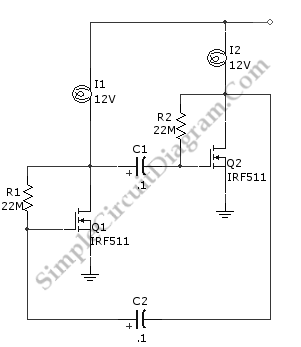# Power FET Lamp Flasher

As an alternative to bipolar transistor, a lamp flasher can be built by using two power FETs.  Like other flasher circuit, this circuit alternately switch the two lamps off and on.  It is based on a simple astable multivibrator that formed by the two power FETs.  To modify the flashing rate, just remember that flash rate is determined by the RC value. Using the value given, the flash rate of this circuit is 1/3Hz. To increase the flash rate, decrease eiter R2 and R1 or C2 and C1. increasing the value of them will slow the flash rate. Here is the schematic diagram of the circuit: